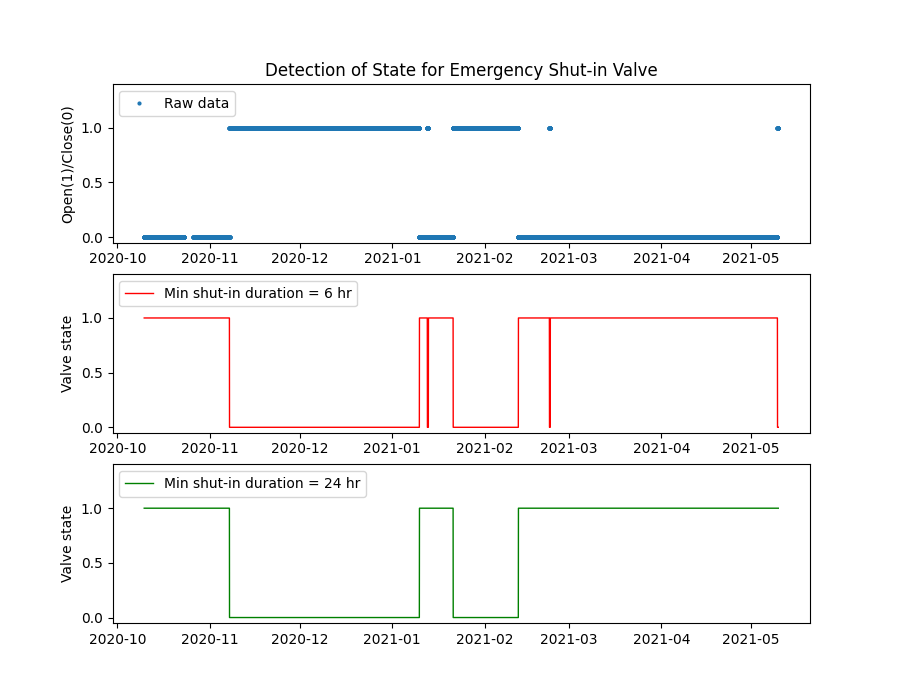# Detection of valve shut-in state

We use data from an emergency shut-down valve sensor on a compressor. The figure shows reading from the valve and the detected open/close state for shut-in durations of at least 6 and 24 hours in duration.```import os

import matplotlib.pyplot as plt
import pandas as pd

from indsl.oil_and_gas.shut_in_detector import calculate_shutin_interval as shi

base_path = "" if __name__ == "__main__" else os.path.dirname(__file__)
data = data.squeeze()
data.index = pd.to_datetime(data.index)
# TODO: Create load_shutin_valve_data method from above

# Apply the shut-in detector with different settings and plot the results
fig, ax = plt.subplots(3, 1, figsize=[9, 7])
ax.plot(data, "o", markersize=2, label="Raw data")
# Short shut-in (default of 6 hrs), shut-in state is when the value is greater than threshold
ax.plot(
shi(data, shutin_state_below_threshold=False),
color="red",
linewidth=1,
label="Min shut-in duration = 6 hr",
)
# Long shut-in (24 hrs), shut-in state is when the value is greater than threshold
ax.plot(
shi(data, min_shutin_len=24, shutin_state_below_threshold=False),
color="green",
linewidth=1,
label="Min shut-in duration = 24 hr",
)
ax.set_ylabel("Open(1)/Close(0)")
ax.set_title("Detection of State for Emergency Shut-in Valve")
ax.set_ylabel("Valve state")
ax.set_ylabel("Valve state")
plt.setp(ax, ylim=[-0.05, 1.4])
_ = ax.legend(loc=2)
_ = ax.legend(loc=2)
_ = ax.legend(loc=2)
plt.show()
```

Total running time of the script: ( 0 minutes 0.300 seconds)

Gallery generated by Sphinx-Gallery